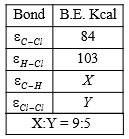You’ve reached the end of your free Videos limit.
#4 | Heat
(Chemistry) > Thermodynamics
Unable to watch the video, please try another server
Related Practice Questions :

Change in enthalpy for reaction,

2H2O2(l)$\to$ 2H2O(l) + O2(g)

if the heat of formation of H2O2(l) and H2O(l) are -188 and -286 kJ/mol respectively is

(a) -196 kJ/mol              (b) + 196 kJ/mol

(c) +948 kJ/mol             (d) -948 kJ/mol

High Yielding Test Series + Question Bank - NEET 2020

Difficulty Level:

If 50 calorie are added to a system and system does work of 30 calorie on surroundings, the change in internal energy of system is:

(a) 20 cal                             (b) 50 cal

(c) 40 cal                             (d) 30 cal

High Yielding Test Series + Question Bank - NEET 2020

Difficulty Level:

Which statements are correct?

(a)  is called Clausius-Clapeyron equation

(b)  is called Trouton's rule

(c) Entropy is a measure of unavailable energy, i.e.,

unavailable energy = entropy x temperature

(d) All of the above

High Yielding Test Series + Question Bank - NEET 2020

Difficulty Level:

The reaction

CH4(g) + Cl2(g)$\to$CH3Cl(g) + HCl(g)

has $∆\mathrm{H}$=-25 K calFrom the given data, what is the bond enthalpy of Cl-Cl bond?

1. 70 K cal                                 2.  80 K cal

3.  67.75 K cal                           4. 57.85 K cal

N2(g) + 3H2(g)$\to$2NH3(g) carried out at constant temperature and pressure. If $∆$H and $∆$E are enthalpy change and internal energy change respectively, which of the following expression is True?